ShanshiashviliB. G.

Georgia, Tbilisi.

Georgian Technical University.

Email: besoshan@hotmajl. corn

IDENTIFICATION OF LINEAR DYNAMICAL SYSTEMS WITH TIME-VARYING PARAMETERS

Abstract. Parameter identification of the linear dynamical systems with time-varying parameters, in case of some restrictions of system's input and output variables and parameters is given. The problem of identification as inverse problem of solution of ordinary linear differential equation with variable coefficients is considered. It is proved theorem about existence of such interval, where the algorithm of the identification of parameters is right.

Шаншиашвили В.Г.

Грузия, Тбилиси.

Грузинский технический университет.

Email; besoshan@hot.mail. corn

ИДЕНТИФИКАЦИЯ ЛИНЕЙНЫХ ДИНАМИЧЕСКИХ СИСТЕМ С ПЕРЕМЕННЫМИ ПАРАМЕТРАМИ

Резюме. Рассматривается задача идентификации параметров линейных динамических систем с переменными параметрами при некоторых ограничениях на входные и выходные переменные и параметры системы. Задача идентификации ставится как обратная задача решения обыкновенного дифференциального уравнения с переменными коеффициентами. Доказывается теорема существования интервала идентификации.

INTRODUCTION

In practice of automatic control linear dynamical systems with time - varying parameters are spread in large. Solution of problems of such system's identification is complicated that mathematical difficulties, which are meeted with solution differential equations with variable coefficients. In particular, by solution of non-homogeneous equations is necessary to known system of fundamental solutions of corresponding to its homogeneous equations, what isn't possible in most cases for real systems. In spite of that there are enough number of papers devoted to the identification of non-stationary systems, in which problems of the identification are solved by different approaches: for instance, by algorithms with approximation by linear combinations of known basic functions , by different modification of recurrent Least Mean Squares identification techniques , by Kalman filtering , by recurrent Bayesian algorithms  and others.

This paper dedicates to investigation of the identification of Imear dynamical systems with time - varying parameters. It means, that it is known structure of system's model. In this case problem of the identification consist in estimation of equations coefficients. Problem of system parameter estimation is considered as inverse problem of solution of ordinary differential equation with variable coefficients, when we have some restriction of system's input and output variables and parameters.

PARAMETER IDENTIFICATION OF LINEAR SYSTEMS

Let's consider multidimensional observed control system with n inputs and n outputs, which is described by following system of normal differential equations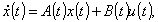(1)

where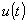and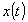are system's input and output vectors,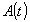and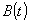are n dimensional quadratic matrices of coefficients.

In this paper the problem of identification is considered in the following way: according to the identification theory , as a result of system's input and output variables observation we found that input variable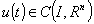and output variables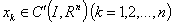, which are solution of the system (1) with different initial state, and also is known matrix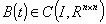, where R is set of real numbers,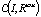- interval,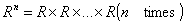,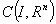and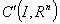- sets of continuous and continuous differentiable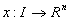functions correspondingly,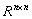- set of n dimensional real quadratic matrices,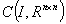- set of all continuous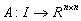matrices. It is required to determine unknown continuous matrix, it means, that it is unknown system of fundamental solutions of system homogeneous equations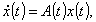(10)

which corresponds to system (1).

Because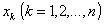is solution of system (1), we have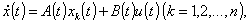(2)

where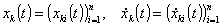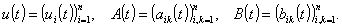Let's introduce matrix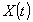, which columns of which are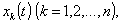: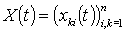and matrix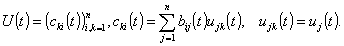Then we can rewrite system (2) like matrix algebraic equation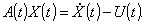(3)

For determination matrix A(t) from (3) it is sufficient, that X(t) matrix is non-degenerated.

Let's show, that there exists such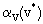, that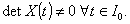Theorem. If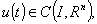and, than there exists intervalsuch that, and in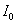matrix A(t) is determined single-valued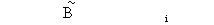(4)

Really, let's admit, thatis solution of system (1) with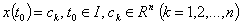(5)

initial condition.

It is known, that solution of problem (1), (5) defined by Cauchy formula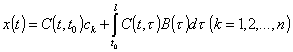(6)

where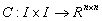matrix function is Cauchy's matrix of system homogeneous equations (1o).

In role of constants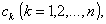we take linearly undependent vectors, in particular: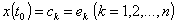(7)

where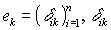- is Kronecker's symbol.

If we consider definition of Cauchy's matrix, we receive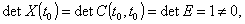(8)

where E - is unity matrix.

Because X(t) is continuous matrix and determinant of continuous matrix is continuous function, therefore there exists interval, such that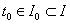and. Therefore for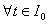there exists inverse matrix and matrix A(t) can be determined by formula (4).

This determination is single-valued because problem of Cauchy (1),(5) for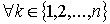has unique solution according to uniqueness and existence theorem.

IDENTIFICATION OF PRACTICAL SYSTEMS

Assume that a system with two inputs and two outputs is described by system of differential equations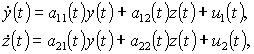(9)

where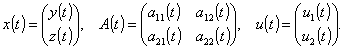Suppose that after input-output system observation it is recieved: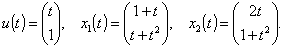(10)

Formmatrix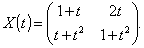(11)

Its determinant is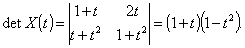(12)

It is clear, that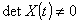, when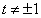. So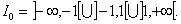(13)

If calculate A(t) matrix by (4) we receive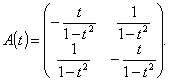(14)

Thus, unknown system is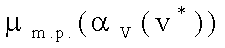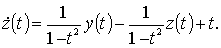(15)

The system of fundamental solutions of system homogeneous equations, which corresponds to system (15), is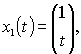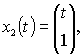(16)

and fundamental matrix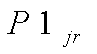(17)

Because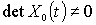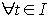, therefore it is clear, that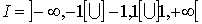(18)

and in this case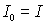.

Thus, for identification of real system, when structure of model is known, we observe system's input and output with different initial states of system. Data, received by observation, we make approximation with continuous functions. Then we compose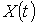matrix and in this interval, where determinant of this matrix differs from zero, we determine the coefficients matrix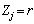by formula (4).

CONCLUSION

In this paper algorithm of the identification of systems parameters is worked out, when we have some restriction of system input and output variables and parameters. It means that model structure of system is known as a priori. The vector of input variables is continuous, and vector of output variables is continuous differentiable. It means, that variable coefficients are continuous. It is proved theorem about existence such interval, where this algorithm is right. In the paper is given example, where is illustrated the use of this algorithm for practical systems. Exactness of parameters estimations is depended on exactness of approximation input-output variables on the basis of data, which are received by observe of system's input- output. To protect from noise we recommend to use least squares method for the approximation input and output variables with continuous function.

REFERENCES

1. Doroslavski M., Fan H. Wavelent - Based Linear System Modeling and Adaptive Filtering// IEEE Trans. Signal Proc. 1996. V. 44. No. 5. P. 1156-1167.
2. Chen Y.-M.., Wu Y.-C. Modified Recursive Least-Squares Algorithm for Parameter Identification// Int. J. Syst. Sci, 1992. V. 23. No. 2. P.187-205.
3. Wang Z.-O., Zhang J. A Kalman Filter Algorithm Using a Moving Window with Applications// Int. J. Syst. Sci., 1995. V 26. No. 8. P. 1465-1478.
4. Havlena V., Kunc D. Bayesian Order Reduction for ARMAX Systems// Proc. 10 th Symp. IFAC on Identification. Copenhagen, 1994. V. 3. P. 1447-1452.
5. EykhoffP. System identification. New York: Willey, 1974.
6. Bibikov lu. N. The course of ordinary differential equations. Moscow: Vicshaia shkola, 1991, (in Russian).

 Site of Information Technologies Designed by  inftech@webservis.ru.